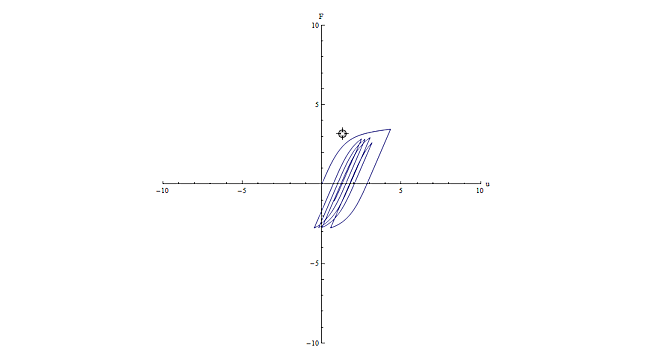#### SDoFBouc-WenmodelwithversioncheckingIntegrating the differential equation of a Bouc-Wen model of hysteresis is very easy using Mathematica. In Mathematica 9, some additional checks prevent NDSolve[ ] from integrating the equation; the error message is NDSolve::smpf: Failure to project onto the discontinuity surface when computing Filippov continuation at time 0.  We can create a version-aware code to take into account this; if the Mathematica version is greater than 8, then remove DiscontinuityProcessing method.

The equation of motion of a single-degree-of-freedom Bouc-Wen system with external linear viscous damping is expressed as:where,is the displacement,is the restoring force,is the excitation force,is the damping coefficient and the overdot denotes the derivative with respect to time. The restoring force is expressed as:where,is the yield force,is the yield displacement,is the ratio of post-yield to pre-yield (elastic) stiffness anda dimensionless hysteretic parameter that obeys the following non-linear differential equation with zero initial conditions:The following example evaluates the response of a single-degree-of-freedom Bouc-Wen model under a sinusoidal excitation, with the addition of sliders and other controls to allow the user to fiddle with the parameters: